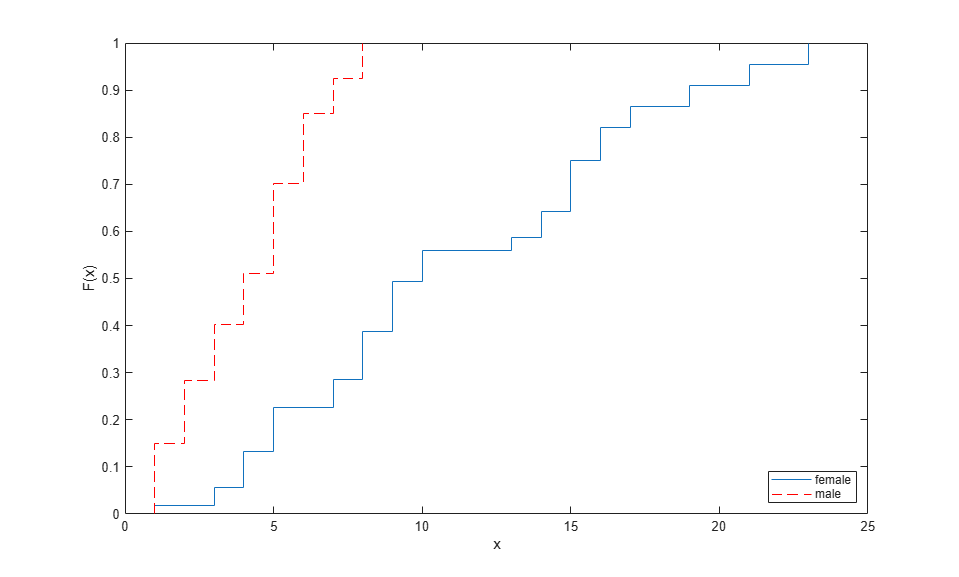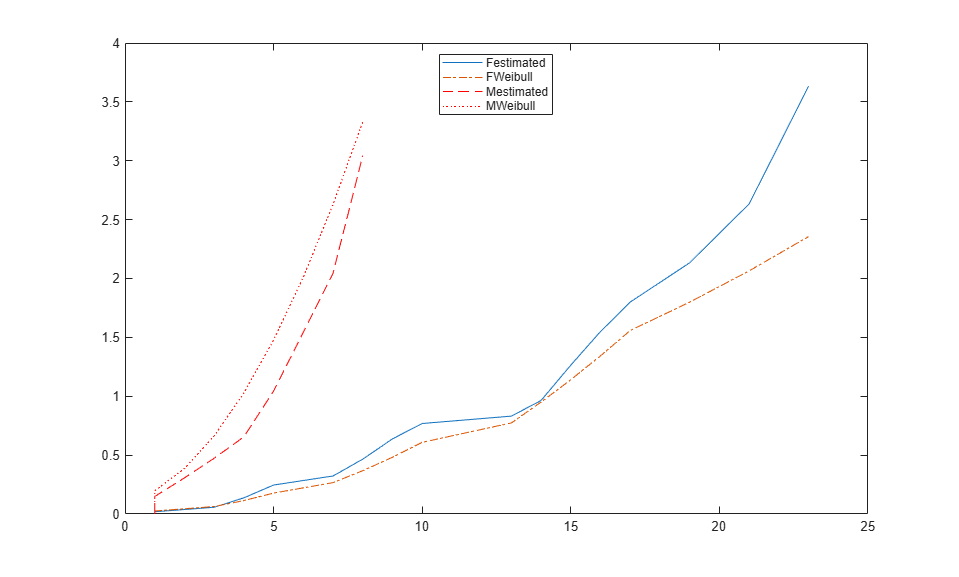# Hazard and Survivor Functions for Different Groups

This example shows how to estimate and plot the cumulative hazard and survivor functions for different groups.

### Step 1. Load and organize sample data.

```load('readmissiontimes.mat') ```

The data has readmission times of patients with information on their gender, age, weight, smoking status, and censorship. This is simulated data.

Create a matrix of readmission times and censoring for each gender.

```female = [ReadmissionTime(Sex==1),Censored(Sex==1)]; male = [ReadmissionTime(Sex==0),Censored(Sex==0)]; ```

### Step 2. Estimate and plot cumulative distribution function for each gender.

Plot the Kaplan-Meier estimate of the cumulative distribution function for female and male patients.

```figure() ecdf(gca,female(:,1),'Censoring',female(:,2)); hold on [f,x] = ecdf(male(:,1),'Censoring',male(:,2)); stairs(x,f,'--r') hold off legend('female','male','Location','SouthEast') ```### Step 3. Plot survivor functions.

Compare the survivor functions for female and male patients.

```figure() ax1 = gca; ecdf(ax1,female(:,1),'Censoring',female(:,2),'function','survivor'); hold on [f,x] = ecdf(male(:,1),'Censoring',male(:,2),'function','survivor'); stairs(x,f,'--r') legend('female','male') ```This figure shows that readmission times are shorter for male patients than female patients.

### Step 4. Fit Weibull survivor functions.

Fit Weibull distributions to readmission times of female and male patients.

```pd = fitdist(female(:,1),'wbl','Censoring',female(:,2)) ```
```pd = WeibullDistribution Weibull distribution A = 12.5593 [10.749, 14.6745] B = 1.99834 [1.56489, 2.55185] ```
```pd2 = fitdist(male(:,1),'wbl','Censoring',male(:,2)) ```
```pd2 = WeibullDistribution Weibull distribution A = 4.63991 [3.91039, 5.50551] B = 1.94422 [1.48496, 2.54552] ```
```pd2 = fitdist(male(:,1),'wbl','Censoring',male(:,2)) ```
```pd2 = WeibullDistribution Weibull distribution A = 4.63991 [3.91039, 5.50551] B = 1.94422 [1.48496, 2.54552] ```

Plot the Weibull survivor functions for female and male patients on estimated survivor functions.

```plot(0:1:25,1-cdf('wbl',0:1:25,12.5593,1.99834),'-.') plot(0:1:25,1-cdf('wbl',0:1:25,4.63991,1.94422),':r') hold off legend('Festimated','Mestimated','FWeibull','MWeibull') ```Weibull distribution provides a good fit for the data.

### Step 5. Estimate cumulative hazard and fit Weibull cumulative hazard functions.

Estimate the cumulative hazard function for the genders and fit Weibull cumulative hazard functions.

```figure() [f,x] = ecdf(female(:,1),'Censoring',female(:,2),... 'function','cumhazard'); plot(x,f) hold on plot(x,cumsum(pdf(pd,x)./(1-cdf(pd,x))),'-.') [f,x] = ecdf(male(:,1),'Censoring',male(:,2),... 'function','cumhazard'); plot(x,f,'--r') plot(x,cumsum(pdf(pd2,x)./(1-cdf(pd2,x))),':r') legend('Festimated','FWeibull','Mestimated','MWeibull',... 'Location','North') ```